Anzeige

# non para.doc

Assistant professor at Annamalai University um Annamalai University
24. Mar 2023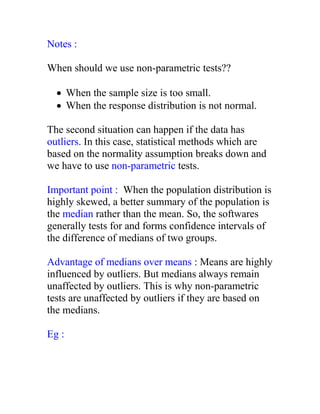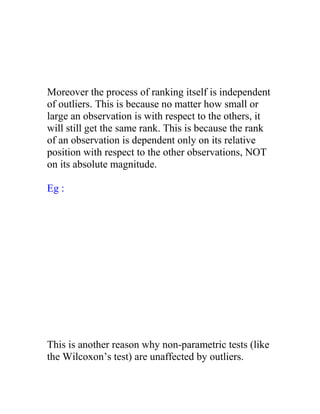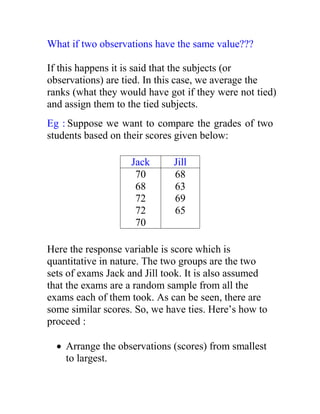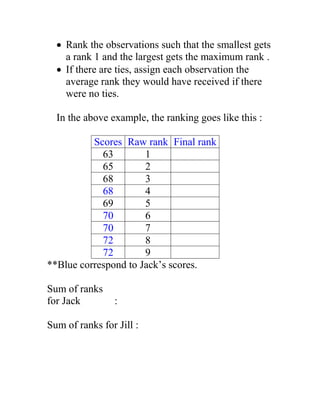Anzeige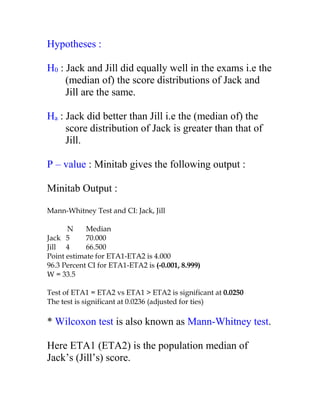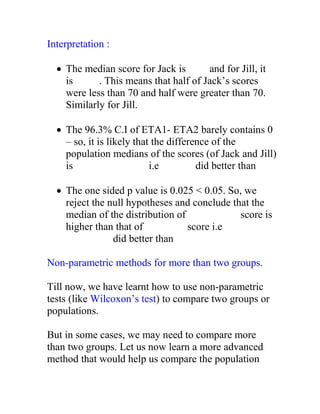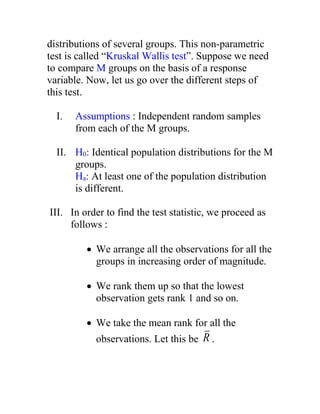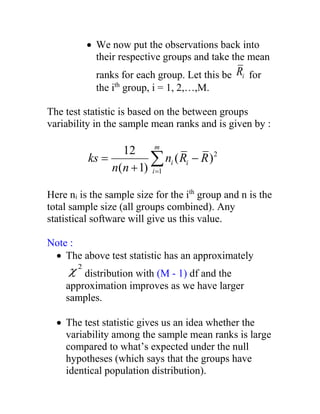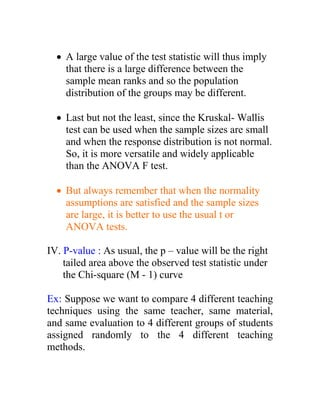Anzeige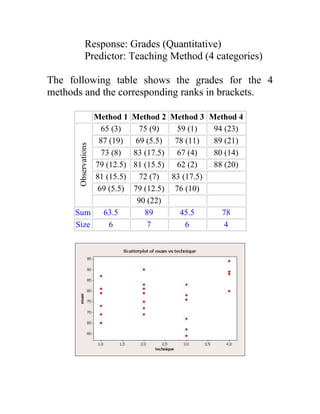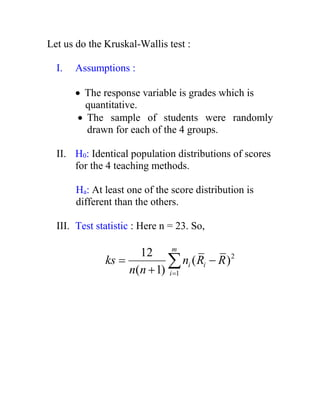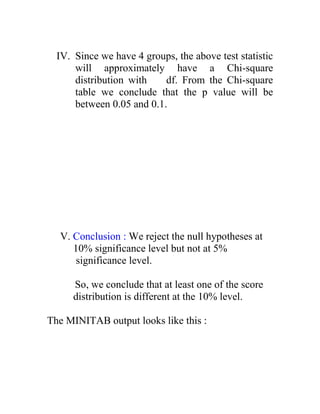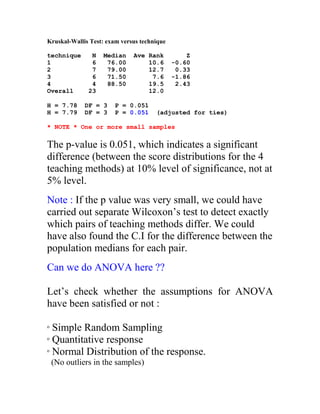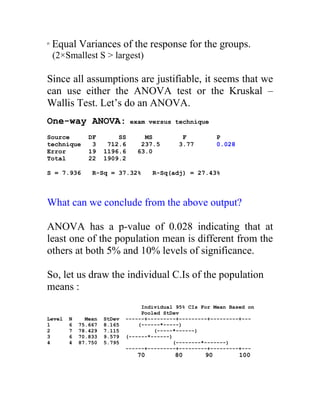Anzeige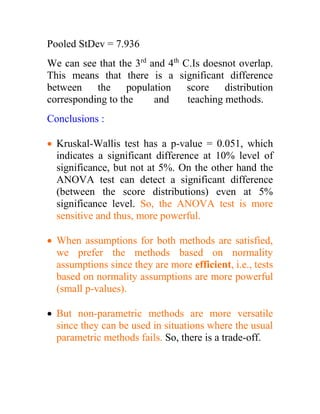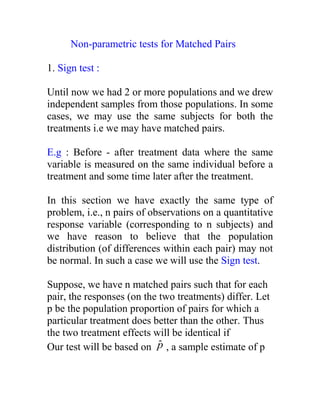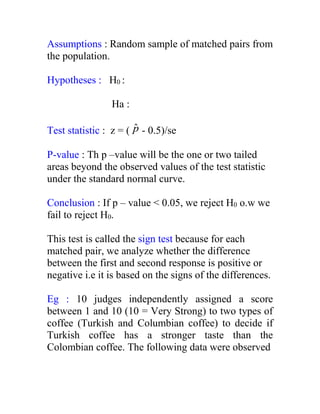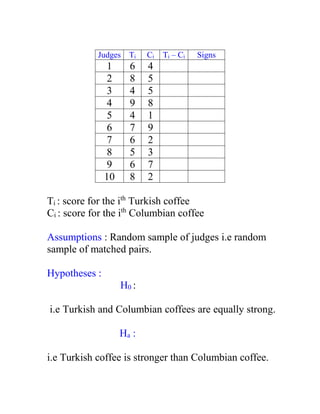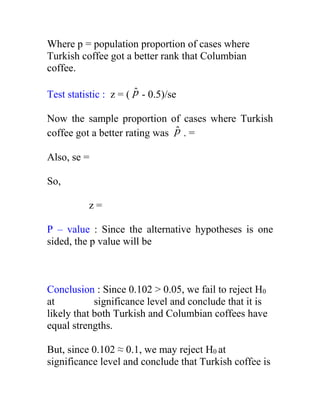AnzeigeNächste SlideShareBasic statistics
1 von 20
Anzeige

### non para.doc

1. Notes : When should we use non-parametric tests??  When the sample size is too small.  When the response distribution is not normal. The second situation can happen if the data has outliers. In this case, statistical methods which are based on the normality assumption breaks down and we have to use non-parametric tests. Important point : When the population distribution is highly skewed, a better summary of the population is the median rather than the mean. So, the softwares generally tests for and forms confidence intervals of the difference of medians of two groups. Advantage of medians over means : Means are highly influenced by outliers. But medians always remain unaffected by outliers. This is why non-parametric tests are unaffected by outliers if they are based on the medians. Eg :
2. Moreover the process of ranking itself is independent of outliers. This is because no matter how small or large an observation is with respect to the others, it will still get the same rank. This is because the rank of an observation is dependent only on its relative position with respect to the other observations, NOT on its absolute magnitude. Eg : This is another reason why non-parametric tests (like the Wilcoxon’s test) are unaffected by outliers.
3. What if two observations have the same value??? If this happens it is said that the subjects (or observations) are tied. In this case, we average the ranks (what they would have got if they were not tied) and assign them to the tied subjects. Eg : Suppose we want to compare the grades of two students based on their scores given below: Jack Jill 70 68 72 72 70 68 63 69 65 Here the response variable is score which is quantitative in nature. The two groups are the two sets of exams Jack and Jill took. It is also assumed that the exams are a random sample from all the exams each of them took. As can be seen, there are some similar scores. So, we have ties. Here’s how to proceed :  Arrange the observations (scores) from smallest to largest.
4.  Rank the observations such that the smallest gets a rank 1 and the largest gets the maximum rank .  If there are ties, assign each observation the average rank they would have received if there were no ties. In the above example, the ranking goes like this : Scores Raw rank Final rank 63 1 65 2 68 3 68 4 69 5 70 6 70 7 72 8 72 9 **Blue correspond to Jack’s scores. Sum of ranks for Jack : Sum of ranks for Jill :
5. Hypotheses : H0 : Jack and Jill did equally well in the exams i.e the (median of) the score distributions of Jack and Jill are the same. Ha : Jack did better than Jill i.e the (median of) the score distribution of Jack is greater than that of Jill. P – value : Minitab gives the following output : Minitab Output : Mann-Whitney Test and CI: Jack, Jill N Median Jack 5 70.000 Jill 4 66.500 Point estimate for ETA1-ETA2 is 4.000 96.3 Percent CI for ETA1-ETA2 is (-0.001, 8.999) W = 33.5 Test of ETA1 = ETA2 vs ETA1 > ETA2 is significant at 0.0250 The test is significant at 0.0236 (adjusted for ties) * Wilcoxon test is also known as Mann-Whitney test. Here ETA1 (ETA2) is the population median of Jack’s (Jill’s) score.
6. Interpretation :  The median score for Jack is and for Jill, it is . This means that half of Jack’s scores were less than 70 and half were greater than 70. Similarly for Jill.  The 96.3% C.I of ETA1- ETA2 barely contains 0 – so, it is likely that the difference of the population medians of the scores (of Jack and Jill) is i.e did better than  The one sided p value is 0.025 < 0.05. So, we reject the null hypotheses and conclude that the median of the distribution of score is higher than that of score i.e did better than Non-parametric methods for more than two groups. Till now, we have learnt how to use non-parametric tests (like Wilcoxon’s test) to compare two groups or populations. But in some cases, we may need to compare more than two groups. Let us now learn a more advanced method that would help us compare the population
7. distributions of several groups. This non-parametric test is called “Kruskal Wallis test”. Suppose we need to compare M groups on the basis of a response variable. Now, let us go over the different steps of this test. I. Assumptions : Independent random samples from each of the M groups. II. H0: Identical population distributions for the M groups. Ha: At least one of the population distribution is different. III. In order to find the test statistic, we proceed as follows :  We arrange all the observations for all the groups in increasing order of magnitude.  We rank them up so that the lowest observation gets rank 1 and so on.  We take the mean rank for all the observations. Let this be R .
8.  We now put the observations back into their respective groups and take the mean ranks for each group. Let this be i R for the ith group, i = 1, 2,…,M. The test statistic is based on the between groups variability in the sample mean ranks and is given by : 2 1 12 ( ) ( 1) m i i i ks n R R n n      Here ni is the sample size for the ith group and n is the total sample size (all groups combined). Any statistical software will give us this value. Note :  The above test statistic has an approximately 2  distribution with (M - 1) df and the approximation improves as we have larger samples.  The test statistic gives us an idea whether the variability among the sample mean ranks is large compared to what’s expected under the null hypotheses (which says that the groups have identical population distribution).
9.  A large value of the test statistic will thus imply that there is a large difference between the sample mean ranks and so the population distribution of the groups may be different.  Last but not the least, since the Kruskal- Wallis test can be used when the sample sizes are small and when the response distribution is not normal. So, it is more versatile and widely applicable than the ANOVA F test.  But always remember that when the normality assumptions are satisfied and the sample sizes are large, it is better to use the usual t or ANOVA tests. IV. P-value : As usual, the p – value will be the right tailed area above the observed test statistic under the Chi-square (M - 1) curve Ex: Suppose we want to compare 4 different teaching techniques using the same teacher, same material, and same evaluation to 4 different groups of students assigned randomly to the 4 different teaching methods.
10. Response: Grades (Quantitative) Predictor: Teaching Method (4 categories) The following table shows the grades for the 4 methods and the corresponding ranks in brackets. Method 1 Method 2 Method 3 Method 4 Observations 65 (3) 75 (9) 59 (1) 94 (23) 87 (19) 69 (5.5) 78 (11) 89 (21) 73 (8) 83 (17.5) 67 (4) 80 (14) 79 (12.5) 81 (15.5) 62 (2) 88 (20) 81 (15.5) 72 (7) 83 (17.5) 69 (5.5) 79 (12.5) 76 (10) 90 (22) Sum 63.5 89 45.5 78 Size 6 7 6 4
11. Let us do the Kruskal-Wallis test : I. Assumptions :  The response variable is grades which is quantitative.  The sample of students were randomly drawn for each of the 4 groups. II. H0: Identical population distributions of scores for the 4 teaching methods. Ha: At least one of the score distribution is different than the others. III. Test statistic : Here n = 23. So, 2 1 12 ( ) ( 1) m i i i ks n R R n n     
12. IV. Since we have 4 groups, the above test statistic will approximately have a Chi-square distribution with df. From the Chi-square table we conclude that the p value will be between 0.05 and 0.1. V. Conclusion : We reject the null hypotheses at 10% significance level but not at 5% significance level. So, we conclude that at least one of the score distribution is different at the 10% level. The MINITAB output looks like this :
13. Kruskal-Wallis Test: exam versus technique technique N Median Ave Rank Z 1 6 76.00 10.6 -0.60 2 7 79.00 12.7 0.33 3 6 71.50 7.6 -1.86 4 4 88.50 19.5 2.43 Overall 23 12.0 H = 7.78 DF = 3 P = 0.051 H = 7.79 DF = 3 P = 0.051 (adjusted for ties) * NOTE * One or more small samples The p-value is 0.051, which indicates a significant difference (between the score distributions for the 4 teaching methods) at 10% level of significance, not at 5% level. Note : If the p value was very small, we could have carried out separate Wilcoxon’s test to detect exactly which pairs of teaching methods differ. We could have also found the C.I for the difference between the population medians for each pair. Can we do ANOVA here ?? Let’s check whether the assumptions for ANOVA have been satisfied or not : o Simple Random Sampling o Quantitative response o Normal Distribution of the response. (No outliers in the samples)
14. o Equal Variances of the response for the groups. (2×Smallest S > largest) Since all assumptions are justifiable, it seems that we can use either the ANOVA test or the Kruskal – Wallis Test. Let’s do an ANOVA. One-way ANOVA: exam versus technique Source DF SS MS F P technique 3 712.6 237.5 3.77 0.028 Error 19 1196.6 63.0 Total 22 1909.2 S = 7.936 R-Sq = 37.32% R-Sq(adj) = 27.43% What can we conclude from the above output? ANOVA has a p-value of 0.028 indicating that at least one of the population mean is different from the others at both 5% and 10% levels of significance. So, let us draw the individual C.Is of the population means : Individual 95% CIs For Mean Based on Pooled StDev Level N Mean StDev ------+---------+---------+---------+--- 1 6 75.667 8.165 (------*-----) 2 7 78.429 7.115 (-----*------) 3 6 70.833 9.579 (------*------) 4 4 87.750 5.795 (--------*-------) ------+---------+---------+---------+--- 70 80 90 100
15. Pooled StDev = 7.936 We can see that the 3rd and 4th C.Is doesnot overlap. This means that there is a significant difference between the population score distribution corresponding to the and teaching methods. Conclusions :  Kruskal-Wallis test has a p-value = 0.051, which indicates a significant difference at 10% level of significance, but not at 5%. On the other hand the ANOVA test can detect a significant difference (between the score distributions) even at 5% significance level. So, the ANOVA test is more sensitive and thus, more powerful.  When assumptions for both methods are satisfied, we prefer the methods based on normality assumptions since they are more efficient, i.e., tests based on normality assumptions are more powerful (small p-values).  But non-parametric methods are more versatile since they can be used in situations where the usual parametric methods fails. So, there is a trade-off.
16. Non-parametric tests for Matched Pairs 1. Sign test : Until now we had 2 or more populations and we drew independent samples from those populations. In some cases, we may use the same subjects for both the treatments i.e we may have matched pairs. E.g : Before - after treatment data where the same variable is measured on the same individual before a treatment and some time later after the treatment. In this section we have exactly the same type of problem, i.e., n pairs of observations on a quantitative response variable (corresponding to n subjects) and we have reason to believe that the population distribution (of differences within each pair) may not be normal. In such a case we will use the Sign test. Suppose, we have n matched pairs such that for each pair, the responses (on the two treatments) differ. Let p be the population proportion of pairs for which a particular treatment does better than the other. Thus the two treatment effects will be identical if Our test will be based on p̂ , a sample estimate of p
17. Assumptions : Random sample of matched pairs from the population. Hypotheses : H0 : Ha : Test statistic : z = ( p̂ - 0.5)/se P-value : Th p –value will be the one or two tailed areas beyond the observed values of the test statistic under the standard normal curve. Conclusion : If p – value < 0.05, we reject H0 o.w we fail to reject H0. This test is called the sign test because for each matched pair, we analyze whether the difference between the first and second response is positive or negative i.e it is based on the signs of the differences. Eg : 10 judges independently assigned a score between 1 and 10 (10 = Very Strong) to two types of coffee (Turkish and Columbian coffee) to decide if Turkish coffee has a stronger taste than the Colombian coffee. The following data were observed
18. Judges Ti Ci Ti – Ci Signs 1 6 4 2 8 5 3 4 5 4 9 8 5 4 1 6 7 9 7 6 2 8 5 3 9 6 7 10 8 2 Ti : score for the ith Turkish coffee Ci : score for the ith Columbian coffee Assumptions : Random sample of judges i.e random sample of matched pairs. Hypotheses : H0 : i.e Turkish and Columbian coffees are equally strong. Ha : i.e Turkish coffee is stronger than Columbian coffee.
19. Where p = population proportion of cases where Turkish coffee got a better rank that Columbian coffee. Test statistic : z = ( p̂ - 0.5)/se Now the sample proportion of cases where Turkish coffee got a better rating was p̂ . = Also, se = So, z = P – value : Since the alternative hypotheses is one sided, the p value will be Conclusion : Since 0.102 > 0.05, we fail to reject H0 at significance level and conclude that it is likely that both Turkish and Columbian coffees have equal strengths. But, since 0.102 ≈ 0.1, we may reject H0 at significance level and conclude that Turkish coffee is
20. stronger than Columbian coffee (if we really donot want to upset the Turks !!).
Anzeige